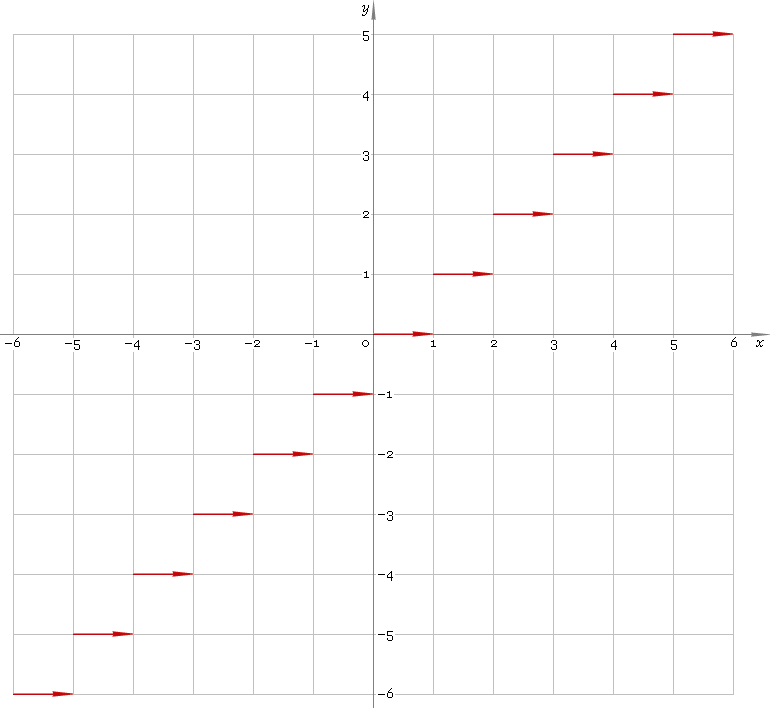The Art of Mathematics

# floor — floor function

## 1. Definition

Floor is the nearest integer to the left — the larget integer less than or equal to the argument.

## 2. Graph

Floor function defined everywhere on real axis — so, its domain is (−∞, +∞). Its stair-like graph is depicted below — fig. 1.Fig. 1. Graph of the floor function y = floorx.

Function codomain is the set of integer numbers.

## 3. How to use

To calculate floor of the number:

``floor(−1.8);``

To get floor of the complex number:

``floor(−1.8+i);``

To get floor of the current result:

``floor(rslt);``

To get floor of the number z in calculator memory:

``floor(mem[z]);``

## 4. Support

Floor function of the complex argument is supported in professional version of the Librow calculator.